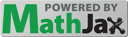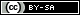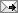## Gavin R. Putland,  BE PhD

 Sunday, January 26, 2014 (Comment)

# Errata for Chapter 1 of Allen & Mills, Signal Analysis: Time, Frequency, Scale, and Structure (IEEE/Wiley, 2004)This page uses MathJax™ to display equations and mathematical symbols. It requires JavaScript™ and an up-to-date browser.

Errors are listed in order of appearance in the book. Errors that I regard as particularly serious are introduced in bold type. The usual disclaimers apply. In particular, I do not guarantee that the list is complete, and I have not attempted to correct all the inconsistencies in fonts (V/$$\boldsymbol{v}$$ ; $$\boldsymbol{x}$$/$$x$$/$${\rm x}$$ ; $$r$$/$${\rm r}$$ ; $$\mathbb{R}$$/).

Page 23, just above §1.2.2: “zero within finite intervals” should be “zero outside finite intervals” (cf. p.64, §1.6.1.2).

Page 24: “contain-san” (with a line break after the hyphen) should be “contains an”.

Page 26, just above §1.2.2.3: “define $$\pi$$ as the unique point $$1\lt \pi/2\lt 2$$, where $$\cos(t)=0$$” should be “define $$\pi/2$$ as the unique point $$t$$ such that $$1\lt t\lt 2$$ and $$\cos t=0$$” or better “define $$\pi$$ as twice the smallest positive $$t$$ such that $$\cos t=0$$”.

Pages 27–8: What the authors call the “Laplace identity” is better known as Euler's formula.

Page 40, §1.4: “Sampling converts an analog signal into a digital signal” should be “Sampling converts an analog signal into a discrete signal”.

Page 47 (end of 1st paragraph): The assertion that “a condition on a polynomial's derivative is much less restrictive than requiring it to contain a given point” is not true in general. Either condition is a single equation to be satisfied. However, requiring a cubic spline to pass through an internal knot (i.e. through a given point other than an endpoint) imposes two conditions: one for the left-hand interpolant and one for the right-hand interpolant; but requiring the left-hand and right-hand derivatives at an internal knot to have the same value — without specifying that value — imposes only one condition.

Page 47 (2nd paragraph): To find $$N$$ cubic interpolants for $$N\!+\!1$$ given points, we must find $$4N$$ coefficients. So we need $$4N$$ equations (although only some of them need to be solved by Gaussian elimination). By requiring the spline to pass through the given points, we get $$2N$$ equations (two equations per interpolant). By requiring the first and second derivatives to match at the $$N\!-\!1$$ internal knots, we get another $$2N\!-\!2$$ equations. Hence we need two more. To obtain them, we can, as the authors say, “specify second derivative values at the endpoints,” but that is not the only option. For example, at one endpoint or both endpoints, we could instead specify the first derivative. This is convenient if (e.g.) the “given” points have been obtained by numerical solution of a differential equation that yields a formula for the derivative. Or, at one end or both ends, we could instead require the third derivatives to match at the nearest internal knot. This is known as the not-a-knot condition because the left-hand and right-hand interpolants are the same cubic. The conditions chosen by the authors, namely that the second derivatives are zero at both endpoints, are known as the natural-spline or free-end conditions, because they imitate the behaviour of a drafter's spline (a flexible rod which can be constrained to pass through given points on a drawing board) when its ends are “free” to assume their “natural” curvature (straight). This choice is algebraically simple, but not necessarily justified by the application.

Page 52: It should be noted (but isn't) that in the equation \begin{equation}\tag{1.57} x(t) = A \cos (2\pi F(t) + \phi)\,, \end{equation} the variable frequency is not $$F(t)$$, but $$F'(t)$$. In the equation \begin{equation}\tag{1.58} x(t) = A \cos (2\pi F + \phi(t))\,, \end{equation} the derivative of $$\phi(t)$$ is the instantaneous radian frequency; hence the instantaneous cycle frequency (in Hz) is not $$F$$, nor $$\phi'(t)$$, but $$\frac{1}{2\pi}\phi'(t)$$.

Page 53: The passage

Over $$\Delta t$$ seconds, the radial frequency of $$x(t)$$ changes by amount $$[\Omega+\phi(t+\Delta t)]-[\Omega+\phi(t)]=\phi(t+\Delta t)-\phi(t)$$, where $$\Omega=2\pi f$$. The average change in Hertz frequency over this time interval is $$\frac{\phi(t+\Delta t)-\phi(t)}{2\pi\,\Delta t}$$.  As $$\Delta t\rightarrow 0\,$$, this value becomes the derivative $$\frac{d}{dt}\phi(t)\,$$, the instantaneous frequency...

should be, at worst,

Over $$\Delta t$$ seconds, the radial phase of $$x(t)$$ changes by amount $$[\Omega+\phi(t+\Delta t)]-[\Omega+\phi(t)]=\phi(t+\Delta t)-\phi(t)$$, where $$\Omega=2\pi F$$. The average Hertz frequency over this time interval is $$\frac{\phi(t+\Delta t)-\phi(t)}{2\pi\,\Delta t}$$.  As $$\Delta t\rightarrow 0\,$$, this value becomes $$\frac{1}{2\pi}\frac{d}{dt}\phi(t)\,$$, the instantaneous frequency...

Pages 54–5, Fig. 1.26 and after Eq.(1.60): No, in general the phase of $$\boldsymbol{v}_1\!+\!\boldsymbol{v}_2$$ is not $$(\phi_2\!-\!\phi_1)/2$$. [Nor is it $$(\phi_2\!+\!\phi_1)/2$$ unless the magnitudes of $$\boldsymbol{v}_1$$ and $$\boldsymbol{v}_2$$ happen to be equal.] But it can be obtained from the $$x$$ and $$y$$ components of the sum.

Page 55, §1.5.2: In property (iii), “the restriction to unit periods or more” implies a maximum radian frequency of $$2\pi$$, not $$\pi$$. However, because a discrete signal with unit period is constant, a discrete periodic sinusoidal signal cannot show any sinusoidal variation unless its period is at least $$2$$. That's why its radian frequency is at most $$\pi$$ (but this is not explained). Hence, in the subsequent “Proposition (Discrete Period)”, the largest frequency for a discrete (periodic) sinusoid should be $$\lvert F\rvert=1/2$$, not $$\lvert F\rvert=1$$. The proof of the “proposition” is relegated to Exercise 11 (p.102, q.v.), which repeats the same error and omission.

Top of page 56: The passage

And, since cosine can only assume the same values on intervals that are integral multiples of $$\pi\,$$, we must have $$\Omega N=m\pi$$ for some $$m\in\mathbb{N}$$. Then, $$\Omega=m\pi/N$$, so that $$\Omega$$ is a rational multiple of $$\pi$$

should be

And, since cosine can only assume the same values on intervals that differ by integral multiples of $$2\pi\,$$, we must have $$\Omega N=2m\pi$$ for some $$m\in\mathbb{N}$$. Then, $$\Omega=2m\pi/N$$, so that $$\Omega$$ is a rational multiple of $$2\pi$$.

Notice that every occurrence of $$\pi$$ should be replaced by $$2\pi$$. (In the last occurrence, a rational multiple of $$\pi$$ is also a rational multiple of $$2\pi$$; but the latter is more to the point.)

Page 64, after “Definition (Finite Support)”: The passage

If $$x(n)$$ is finitely supported, then it can be specified via square brackets notation: $$x=[k_M,\ldots,\underline{kd_0},\ldots,k_N]$$, where $$x(n)=k_n$$ and $$M\leq 0\leq N$$

should be, at worst,

If $$x(n)$$ is finitely supported with $$M\leq 0\leq N$$, then it can be specified via square brackets notation: $$x=[k_M,\ldots,\underline{k_0},\ldots,k_N]$$, where $$x(n)=k_n$$.

Page 65, last line: “absolutely summability” should be “absolute summability”.

Page 66, after Eq.(1.66): “Signals that are integrable of an interval...” should be “Signals that are absolutely integrable on an interval...”.

Page 66, before Eq.(1.70): Signals that satisfy \begin{equation}\tag{1.70} \int_a^b \! \lvert x(t)\rvert^2 dt \lt \infty \end{equation} are called $$L^2[a,b]$$ signals, not $$L^1[a,b]$$ signals.

Top of page 67: “the sum of the squares of its electric and magnetic fields” should be “a weighted sum of the squares of its electric and magnetic fields”. The squares can't be added directly, because their dimensions (units) don't match.

Page 73, “Theorem (Cauchy-Riemann Equations)”: The second equation should be \begin{equation}\tag{1.82b} \frac{\partial u}{\partial y}(w)= - \frac{\partial v}{\partial x}(w). \end{equation} That is, it should have a minus sign in it, as can be shown by equating the imaginary parts of Eqs. (1.84a) and (1.84b) on p.74.

Page 76: Eq.(1.87) should be \begin{equation}\tag{1.87} \int\limits_C f(z)\,dz = \!\int_a^b \! f\big(s(t)\big)\,s'(t)\,dt\,, \end{equation} where $$s(t)$$, for $$t\in[a,b]$$, parameterizes $$C$$. Note that the first integral sign should not have a ring (because a ring indicates a closed loop, but here we want $$C$$ to be an open arc).

Page 76, “Theorem (Cauchy Integral for a Circle)”: Eq.(1.88) omits a minus sign on the top line. The integrand in that equation is not necessarily analytic, and its connection with $$f(z)$$ is not explained. The theorem should say [quote]: If $$f(z)=z^m$$ (where $$m\in\mathbb{N}$$) in a region containing the closed circle $$C$$, with radius $$R$$ and center $$(0,0)$$, traversed counterclockwise, then \begin{equation}\tag{1.88} \frac{1}{2\pi j}\oint\limits_C f(z)\,dz = \begin{cases} 0 & \text{if } m\neq -1\,,\\ 1 & \text{if } m=-1\,. \end{cases} \end{equation} [Unquote]. On the next page, after Eq.(1.91), the proof should say: “Now let $$f(z)=z^m$$. Suppose $$m=-1$$,” etc.

Page 78, “Definition (Residue)”: Eq.(1.95) is wrong (see below). The residue of $$f(z)$$ at a pole $$z\!=\!p$$ may be defined as \begin{equation}\label{eres20140126} {\rm Res}\big(f(z),p\big)=\frac{1}{2\pi j}\oint\limits_C f(z)\,dz\,, \end{equation} where $$C$$ is a counterclockwise simple closed contour enclosing that pole and no others. From this definition and the corrected Eq.(1.88) and the Cauchy Integral Theorem, it can be shown that the residue is the coefficient of $$(z\!-\!p)^{-1}$$ in the Laurent (power series) expansion of $$f(z)$$ about $$p$$. Hence it can be shown that \begin{equation}\label{ereslim20140126} {\rm Res}\big(f(z),p\big) = \frac{1}{(k\!-\!1)!} \frac{d^{k-1}}{dz^{k-1}} \left[(z\!-\!p)^k\,f(z)\right]_{z\rightarrow p}\,, \end{equation} where $$k$$ is the order of the pole.

Page 78, “Theorem (Cauchy Residue)”: The equation should be \begin{equation}\tag{1.96} \frac{1}{2\pi j}\oint\limits_C \frac{f(z)}{(z\!-\!a)^m}\,dz = \begin{cases} \frac{1}{(m-1)!} f^{(m-1)}(a) & \text{if } a \text{ is inside } C\,,\\[1ex] 0 & \text{if } a \text{ is outside } C. \end{cases} \end{equation} that is, the exponent in the denominator on the left should be $$m$$, not $$m\!-\!1$$. The corrected result may be derived as follows. Suppose that $$f(z)$$ is analytic on and inside a counterclockwise simple closed contour $$C$$, and that $$f(a)$$ is finite and nonzero. If $$a$$ is outside $$C$$, then the integrand on the left-hand side is analytic inside $$C$$, so the integral is zero. If $$a$$ is inside $$C$$, then the integrand has a pole of order $$m$$ at $$z\!=\!a$$, and the whole left-hand side is the residue of the integrand at that pole. The residue may be found by writing $$m$$ for $$k$$, and $$a$$ for $$p$$, and $$f(z)/(z\!-\!a)^m$$ for $$f(z)$$, in Eq.($$\ref{ereslim20140126}$$).

So, in Eq.(1.95) on page 78, on the right-hand side, the authors have actually given the residue of $$f(z)/(z\!-\!p)^k$$ at $$p$$, if $$f(z)$$ is analytic on and inside $$C$$ and is finite and nonzero at $$z\!=\!p$$. Which is not what they say on the left-hand side. And they haven't yet defined $$C$$ in this context.

Page 80, “Definition (Algebra and σ-Algebra)”: “$$\wp(\Sigma)$$” should be “$$\wp(\Omega)$$”, i.e. the set of all subsets of $$\Omega$$. It should be made clear at the outset that $$\Sigma$$ is the algebra, not a member set thereof; e.g., “An algebra $$\Sigma$$ over a set $$\Omega$$ is a set of subsets of $$\Omega$$ with the following properties:” etc. Why is this definition useful for present purposes? Because if the member sets of $$\Sigma$$ are called events (as in probability theory) then (i) the empty set is an event, and (ii) if set $$A$$ is an event, its complement (“not” $$A$$) is an event, and (iii) if sets $$A$$ and $$B$$ are events, their union (event $$A$$ “or” event $$B$$) is an event; and from these properties we can show that if sets $$A$$ and $$B$$ are events, their intersection (event $$A$$ “and” event $$B$$) is an event; etc.

Page 82, “Definition (Independent Events)”: The factor $$P(B)$$ should be omitted; that is, $$A$$ and $$B$$ are independent if $$P(A\lvert B)=P(A)$$. From this definition and Eq.(1.101), it follows that $$A$$ and $$B$$ are independent if $$P(A\cap B)=P(A)\,P(B)$$.

Page 84, in “Definition (Random Variable)” and the subsequent “Notation”: “$$\omega\in\mathbb{R}$$” should be “$$\omega\in\Omega$$”, because the domain of the function $$x$$ is said to be $$\Omega$$, not $$\mathbb{R}$$ (cf. Problem 24 on p.104). The definition may be restated thus: A real random variable on a sample space $$\Omega$$ is a function $$x$$, mapping $$\Omega$$ to the real numbers, such that if $$r$$ is real, then “$$x\leq r$$” is an event.

Page 86, line 4: Each occurrence of “$$[r_m,r_n)$$” should be “$$[r_n,r_{n+1})$$”.

Page 86, before Eq.(1.111): “$$P(r_n\leq x\leq r_{n+1})$$” should be “$$P(r_n\leq x\lt r_{n+1})$$”, i.e. the probability that $$x\in[r_n,r_{n+1})$$.

Page 86, “Definition (Discrete Density Function)”: Again “$$[r_m,r_n)$$” should be “$$[r_n,r_{n+1})$$”. But, as the value of $$F_x(r)$$ changes between $$r\!\lt\!r_n$$ and $$r\!=\!r_n$$ (not between $$r\!=\!r_n$$ and $$r\!\gt\!r_n$$), the equation should be \begin{equation}\tag{1.112} f_x(r) = \begin{cases} F_x(r_n)-F_x(r_{n-1}) & \text{if } r=r_n\,,\\ 0 & \text{otherwise}. \end{cases} \end{equation} Hence $$f_x(r)$$ has the property \begin{equation} F_x(r)=\sum_n\,f_x(r_n)\,u(r\!-\!r_n)\ \end{equation} (cf. Fig.1.34, p.87).

Page 91, line 8: At the left-hand end of the line, “$$p(\boldsymbol{v}\lvert C_k)$$” is apparently correct, as it agrees with Eq.(1.125); but in the middle of the line, “$$P(\boldsymbol{v}\lvert C_k)$$” should be “$$P(C_k\lvert\boldsymbol{v})$$”.

Page 91, before Eq.(1.125): “$$P(\boldsymbol{v}\lvert C_k)$$” should be “$$p(\boldsymbol{v}\lvert C_k)$$” in order to agree with the equation.

Page 91, “Definition (Random Signal)”: “$$\boldsymbol{X}=\{x(r):t\in T\}$$” should be “$$\boldsymbol{X}=\{x(t):t\in T\}$$” or perhaps “$$\boldsymbol{X}=\{x(r):r\in T\}$$”, so that the index of $$x$$ is a member of $$T$$.

Page 102, Problem 11: “$$\lvert F\rvert=1$$” should be “$$\lvert F\rvert=1/2$$”; see above concerning Page 55, §1.5.2.

Page 103, Problem 16(f): In this context, “norm” means modulus or absolute value.

Page 105, Problem 27: No, there is not a unique discrete sinusoid with a given radial frequency, because the amplitude and phase are undetermined. But for a given real discrete sinusoid, there is a unique radial frequency $$\omega$$ in the range $$0\leq\omega\leq\pi$$.TweetReturn to Contents